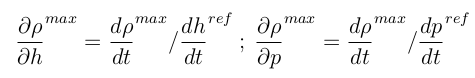# Truncation Method

This strategy acts on the terms ∂ρ/∂p and ∂ρ/∂h. The peak in the density derivative occurring after the transition from liquid to two-phase is truncated, as shown in the figure below. The maximum time derivative of the density is a model parameter and the maximum partial derivatives are calculated using the following equation:This strategy conserves mass balance in each cell, except when a phase transition occurs. In this case, a fictitious creation or destruction of mass appears. The error of this method on the simulation results and on the heat balances can be significant. It should therefore be used with care.

This approach can be implemented at the Modelica level, i.e. it does not involve a modification of the equation of state.

# Reference

Sylvain Quoilin, Ian Bell, Adriano Desideri, and Vincent Lemort. Methods to increase the robustness of finite-volume flow models in thermodynamic systems. To be published in Energies, 2014.